# The MDC Procedure

### Example 18.4 Testing for Homoscedasticity of the Utility Function

The conditional logit model imposes equal variances on random components of utility of all alternatives. This assumption can often be too restrictive and the calculated results misleading. This example shows several approaches to testing the homoscedasticity assumption.

The section Getting Started: MDC Procedure analyzes an HEV model by using Daganzo’s trinomial choice data and displays the HEV parameter estimates in Figure 18.15. The inverted scale estimates for mode "2" and mode "3" suggest that the conditional logit model (which imposes equal variances on random components of utility of all alternatives) might be misleading. The HEV estimation summary from that analysis is repeated in Output 18.4.1.

Output 18.4.1: HEV Estimation Summary (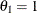)

Model Fit Summary
Dependent Variable decision
Number of Observations 50
Number of Cases 150
Log Likelihood -33.41383
Maximum Absolute Gradient 0.0000218
Number of Iterations 11
Optimization Method Dual Quasi-Newton
AIC 72.82765
Schwarz Criterion 78.56372

You can estimate the HEV model with unit scale restrictions on all three alternatives (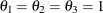) with the following statements.

/*-- HEV Estimation --*/
proc mdc data=newdata;
model decision = ttime /
type=hev
nchoice=3
hev=(unitscale=1 2 3, integrate=laguerre)
covest=hess;
id pid;
run;


Output 18.4.2 displays the estimation summary.

Output 18.4.2: HEV Estimation Summary ()

The MDC Procedure

Heteroscedastic Extreme Value Model Estimates

Model Fit Summary
Dependent Variable decision
Number of Observations 50
Number of Cases 150
Log Likelihood -34.12756
Maximum Absolute Gradient 6.7951E-9
Number of Iterations 5
Optimization Method Dual Quasi-Newton
AIC 70.25512
Schwarz Criterion 72.16714

The test for scale equivalence (SCALE2=SCALE3=1) is performed using a likelihood ratio test statistic. The following SAS statements compute the test statistic (1.4276) and its p-value (0.4898) from the log-likelihood values in Output 18.4.1 and Output 18.4.2:

data _null_;
/*-- test for H0: scale2 = scale3 = 1 --*/
/*  ln L(max) = -34.1276                */
/*  ln L(0)   = -33.4138                */
stat = -2 * ( - 34.1276 + 33.4138 );
df = 2;
p_value = 1 - probchi(stat, df);
put stat= p_value=;
run;


The test statistic fails to reject the null hypothesis of equal scale parameters, which implies that the random utility function is homoscedastic.

A multinomial probit model also allows heteroscedasticity of the random components of utility for different alternatives. Consider the utility function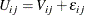where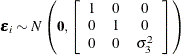This multinomial probit model is estimated by using the following statements:

/*-- Heteroscedastic Multinomial Probit --*/
proc mdc data=newdata;
model decision = ttime /
type=mprobit
nchoice=3
unitvariance=(1 2)
covest=hess;
id pid;
restrict RHO_31 = 0;
run;


The estimation summary is displayed in Output 18.4.3.

Output 18.4.3: Heteroscedastic Multinomial Probit Estimation Summary

The MDC Procedure

Multinomial Probit Estimates

Model Fit Summary
Dependent Variable decision
Number of Observations 50
Number of Cases 150
Log Likelihood -33.88604
Log Likelihood Null (LogL(0)) -54.93061
Maximum Absolute Gradient 5.60277E-6
Number of Iterations 8
Optimization Method Dual Quasi-Newton
AIC 71.77209
Schwarz Criterion 75.59613
Number of Simulations 100
Starting Point of Halton Sequence 11

Next, the multinomial probit model with unit variances (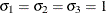) is estimated in the following statements:

/*-- Homoscedastic Multinomial Probit --*/
proc mdc data=newdata;
model decision = ttime /
type=mprobit
nchoice=3
unitvariance=(1 2 3)
covest=hess;
id pid;
restrict RHO_21 = 0;
run;


The estimation summary is displayed in Output 18.4.4.

Output 18.4.4: Homoscedastic Multinomial Probit Estimation Summary

The MDC Procedure

Multinomial Probit Estimates

Model Fit Summary
Dependent Variable decision
Number of Observations 50
Number of Cases 150
Log Likelihood -34.54252
Log Likelihood Null (LogL(0)) -54.93061
Maximum Absolute Gradient 1.37303E-7
Number of Iterations 5
Optimization Method Dual Quasi-Newton
AIC 71.08505
Schwarz Criterion 72.99707
Number of Simulations 100
Starting Point of Halton Sequence 11

The test for homoscedasticity (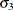= 1) under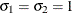shows that the error variance is not heteroscedastic since the test statistic (1.313) is less than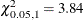. The marginal probability or p-value computed in the following statements from the PROBCHI function is 0.2519:

data _null_;
/*-- test for H0: sigma3 = 1 --*/
/*   ln L(max) = -33.8860      */
/*   ln L(0)   = -34.5425      */
stat = -2 * ( -34.5425 + 33.8860 );
df = 1;
p_value = 1 - probchi(stat, df);
put stat= p_value=;
run;